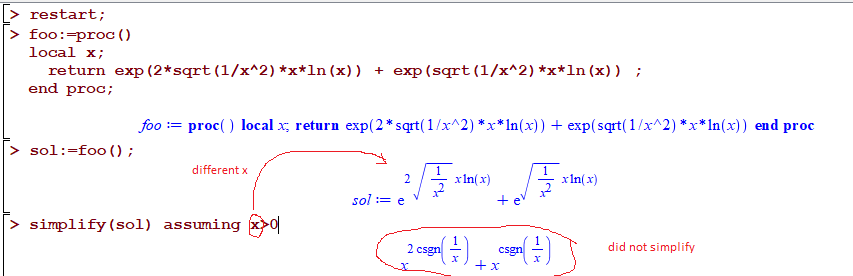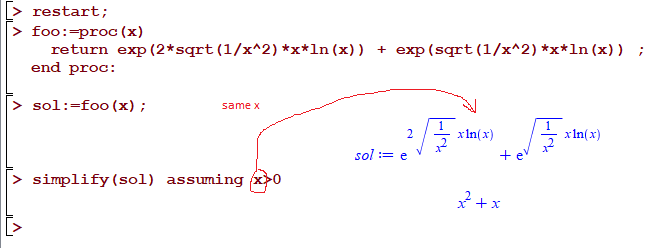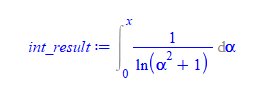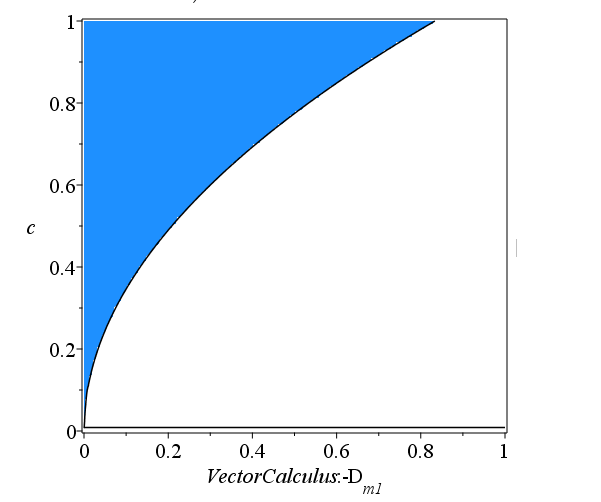## Female Escorts in Aerocity

by: Maple

If all of you visit our official website then you will know our adorable Aerocity escorts that what type services all of her offer you. Our all female escorts in Aerocity so honest, enjoyable, cooperative and fully experienced with her profession. Book sexy Aerocity escorts services and get full untouched bodily entertainment.

## Maple - river flow problem ...Not sure part A is done correctly. flow (m^3 / s) = Area (M^2) * velocity (m/s)

And have no idea how to do part B in maple

Thank you!

## Maple matrix operation and system equation solving...How to solve the system equation with Matrix? Basically is part (b)

And not quite familiar with Matrix multiplication in maple? How to do part(c)?

Thank you!!!!

## What is the best curve fit for Primes of the form ...

Hi all,

We want to find a curve fit for an integer sequence.

We have n such that n^2+n+17 is a prime number.

Use the Maple CurveFitting package.

I tried with(CurveFitting).

We do not know if this is best represented by a polynomial or exponential curve fit.

n2_and_n_and_17_in_OEIS_007635.mw

n2_and_n_and_17_in_OEIS_007635.pdf

Regards,

Matt

## What Happened to the Old Low-level Command "RTABLE...

The low-level command is "RTABLE". I have used it a million times. I use it with Maple 13 to replace expressions by tables.
For example,

print(`Hello! `*RTABLE(1,x^2+y^2));

will print: Hello! x^2+y^2. However, the main reason I am using it is to avoid Maple messing up the order of things like sums,
products, etc. However, this command has disappeared. It does not exist in Maple 2020. So, I cannot run my programs at the university which now has Maple 2020. Anybody knows if this command can still be used in another form the same way?

Thank you!
mapleatha

## Maple Conference - It's Almost Here!

by: Maple

The 2020 Maple Conference is coming up fast! It is running from November 2-6 this year, all remotely, and completely free.

The week will be packed with activities, and we have designed it so that it will be valuable for Maple users of all skill and experience levels. The agenda includes 3 keynote presentations, 2 live panel presentations, 8 Maplesoft recorded presentations, 3 Maple workshops, and 68 contributed recorded presentations.

There will be live Q&A’s for every presentation. Additionally, we are hosting what we’re calling “Virtual Tables” at every breakfast (8-9am EST) and almost every lunch (12-1 EST). These tables offer attendees a chance to discuss topics related to the conference streams of the day, as well as a variety of special topics and social discussions. You can review the schedule for these virtual tables here.

Attendance is completely free, and we’re confident that there will be something there for all Maple users. Whether you attend one session or all of them, we’d love to see you there!

## Particularsol doesn't work properly ...Hello! I would like to ask a short question about particularsol function. Could someone please explain to me, why the function interprets the cosinus term as particular solution, even though it is homogeneous solution? Is it a bug in Maple or I unterstand something wrong?

## Maple is unable to solve this integral. Help!!!...

I am trying to find the surface area of f(x,y) but Maple seems unable to calculate it even numerically.  Any help would be much appreciated.  I'm not sure if I have written this correctly.

f := (x, y) -> x^4*sin(x^2 + y^3)^2*ln(y) + 2*y*x

fx := 4*x^3*sin(y^3 + x^2)^2*ln(y) + 4*x^5*sin(y^3 + x^2)*ln(y)*cos(y^3 + x^2) + 2*y

fy := 6*x^4*sin(y^3 + x^2)*ln(y)*y^2*cos(y^3 + x^2) + x^4*sin(y^3 + x^2)^2/y + 2*x

Int(Int(sqrt(fx^2 + fy^2 + 1.), x = 0. .. 4.), y = 1. .. 5.)

## Better way to simplify expressions returned from p...

What is the best way to handle this?  Many times one wants to return an expression from inside a proc, which uses local symbls, back to the user (global context).

The problem is, if one tries to simplify this returned expression, adding assumptions on some of the symbols in the expression, it does not work. Since the symbol used in the assuming is global, while the symbol inside the returned expression was local to the proc.

Even though the symbols look the same on the screen, they are actually different symbols, so the simplify does not work as expected.

Here is a simple example

```restart;
foo:=proc()
local x;
return exp(2*sqrt(1/x^2)*x*ln(x)) + exp(sqrt(1/x^2)*x*ln(x)) ;
end proc;

sol:=foo();

simplify(sol) assuming x>0```

The above does not work. Since the "x" in assuming x>0 is global, while the "x" in the expression was local to the proc. So even though they look same, they are different symbols.The standard way to handle this, is to pass the "x" to be used to build the solution, from the user to the proc(), so that when the expression is returned, the "x" used will be the global one. Like this

```restart;
foo:=proc(x)
return exp(2*sqrt(1/x^2)*x*ln(x)) + exp(sqrt(1/x^2)*x*ln(x)) ;
end proc;

sol:=foo(x);

simplify(sol) assuming x>0
```

Now it works:But this method is not practical all the time. Suppose the local proc wants to generate an expression with other symbols in it, that the user does not know about. Say alpha, beta, z, eta, and so on. The user does not care about having to pass every possible symbol down on each call.

Is there a way to tell assuming, that the symbols in assumptions command, are to be taken from the expression itself, and not to be global ones?    i.e. when doing

`simplify(sol) assuming x>0`

I want Maple to take that "x" in assuming to be the "x" inside the expression only, and not a global "x".

This will make life much simpler.  I remember seeing other use of assuming where this could be done, but I can't find it yet.

edit:

This is  just one example, where returning expression with new symbol from local proc can be required sometimes.

This is similar to using constant of integrations _C1 by dsolve when it returns a solution.

But those _C1 are all predefined as system/global symbols. But there can be cases where one needs to use new symbols.

An example is where int() timesout or it does not produce result.

In this case, instead of leaving it as is (since I need to use the result and do not want Maple to keep evaluating it in the expression it is in), so  I replace int(integrand,x) with Int( new_integrand , alpha=0...x) where new_integrand is the same as integrand but with each in it, is replaced by new symbol alpha

This symbol alpha has to be local to the proc (it was not passed down by user).

Maple uses _Z sometimes for such a cases, which I do not like. (it looks bad in Latex)

Here is an example

```foo:=proc(x)
local int_result,alpha;
local integrand:=1/ln(x^2+1);

int_result:= int(integrand,x);
if has(int_result,'int') then  #did not integrate
int_result:=Int(subs(x=alpha,integrand),alpha=0..x);
fi;

return int_result;
end proc:

int_result:=foo(x)
```The expression returned contains symbol alpha, which is local to the proc.  Only way around this, is to have the user pass in alpha as well as x, just in case it is needed, which is not practical to do.

This is just one example of many. Another example is when doing some transformation internally (change of variables) to convert the ode from one form to another, and I need to return those intermediate results back to caller. These substitutions use local symbols.

As I said, I could have used _Z or some other system/global known symbol for this. But I do not like how this looks in Latex. And if I need another symbol for another case, I have to look for another one.  Instead, I just make my own local symbols and use them in the expression. (Except for constant of integrations, I use those _C1,_C2, etc....

## How to conver a list of strings to a list of vecto...

Hi friends,

I have the next list of strings and I want to convert it to a list of vectors.

with(StringTools)

Generate(3, "012");
["000", "001", "002", "010", "011", "012", "020", "021", "022",

"100", "101", "102", "110", "111", "112", "120", "121", "122",

"200", "201", "202", "210", "211", "212", "220", "221", "222"]

Or maybe there's an easiest way to generate all the n-ary vectors of a given lenght.

## Evaluate an integral...

Dear all

I need your help to evaluate the following integral using Maple

int(x^x,x=0..1)

Thank you for any help

## Convert digits of numbers into Arrays...

Dear friends,

I have a given number and I need to convert its digits into an array. For example:

a:= 456;  and I need an array [4 5 6].

I know how to obtain such a procedure as a list

map(parse, StringTools:-Explode(convert(a, string)));

but not how to get the result as an array. Could you please help me with the right commands?

Many thanks for the help.

## searchtext result position...

I think  the help file regarding searchtext should be more specific regarding the result of the search.

Using searchtext(pattern, string, range), the result will be an integer which indicates the position of the first character from the beginning of the range - not from the beginning of the string.

One of the examples actually shows that.

SearchText("ijklm", "abcdefghijklmnopqrstuvWxy", 5..-5);

## label display issue...

`inequal({Re(ecs1) <= c, Re(ecs2) <= c, Re(ecs3) <= c}, Dm1 = 0 .. 1, c = 0 .. 1, color = "DodgerBlue", numpoints = 8000, transparency = 0, labels = [D[m1], c], axesfont = [16, 16], labelfont = [16, 16], axes = boxed)`

Hi there,

I have this issue when I try to name my label to Dm1 I get back "VectorCalculus:-Dm1". I know that after I have the figure, I can manually change it but I have many of them and want to save them automatically, therefore it would be necessary to immediately get the right label.

Are there any suggestions? Maybe a different way, how to write subscript?## why DEtools:-particularsol hangs when dsolve works...

I solved this linear constant coefficients ode by hand, and wanted to use Maple to check my solution.

dsolve works with no problem. I wanted to check the particular solution on its own, so called DEtools:-particularsol(ode,y(x)); but was surprised it hangs.

Why would DEtools:-particularsol(ode,y(x)); hang, when dsolve gives the complete solution (including particular solutin ofcourse) right away?  It is 7th order ODE. But nothing special. Linear and constant coefficients. Since dsolve solves it right away, I expected DEtools:-particularsol to have no problem as well.

```restart;
ode:=diff(y(x),x\$7)-2*diff(y(x),x\$6)+9*diff(y(x),x\$5)-16*diff(y(x),x\$4)+24*diff(y(x),x\$3)-32*diff(y(x),x\$2)+16*diff(y(x),x)=exp(2*x)+x*sin(x)+x^2;

dsolve(ode);  #no problem.

#this hangs. Why?
DEtools:-particularsol(ode,y(x));```

 > interface(version);> Physics:-Version()> restart; ode:=diff(y(x),x\$7)-2*diff(y(x),x\$6)+9*diff(y(x),x\$5)-16*diff(y(x),x\$4)+24*diff(y(x),x\$3)-32*diff(y(x),x\$2)+16*diff(y(x),x)=exp(2*x)+x*sin(x)+x^2;> #this works sol:=dsolve(ode)> #this hangs. Why? DEtools:-particularsol(ode,y(x));
 >

 1 2 3 4 5 6 7Last Page 1 of 1738